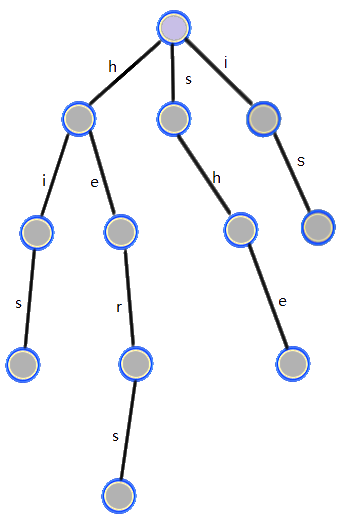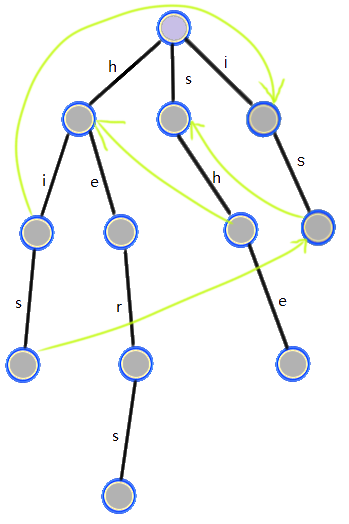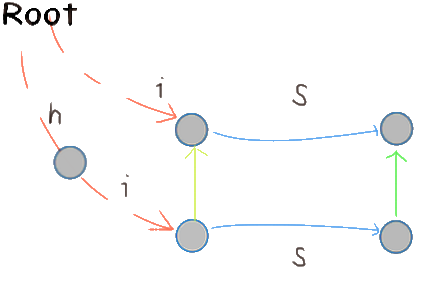# AC自动机学习笔记

Posted by Panda2134's Blog on March 28, 2018

## Trieint sz, val[MAX_NODE+10], ch[MAX_NODE+10][SIGMA+10];
std::unordered_map<string, int> ms;
inline int idx(int c) { return c - 'a' + 1; }
void insert(char *str) {
int len = strlen(str), u = 0;
for(int i = 0; i < len; i++) {
int c = idx(str[i]);
// 建立新点，再向前走一步
if(!ch[u][c]) ch[u][c] = ++sz;
u = ch[u][c];
}
++val[u];
ms[string(str)] = u;
}


## AC 自动机

1. 某个模板串 $T_i$ 的后缀是另一个模板串 $T_j$ 的前缀。这样的话匹配完成 $T_i$ 后， $T_j$ 也完成了部分的匹配，应该跳到 $T_j$ 看匹配完成没有。如 $T_i = \mathtt{“his”}, T_j = \mathtt{“she”}$ 。
2. 某个模板串失配了，但是可能匹配上前缀相同的另一模板。如 $\mathtt{“his”}$ 和 $\mathtt{“hers”}$ 。

1. 某个模板串 $T_i$ 的后缀等于另一个模板串 $T_j$ 。如 $\mathtt{“his”}$ 和 $\mathtt{“is”}$ 。

### 失配边

• 对于 1 ，从 $T_i$ 的结尾点向 $T_j$ 的相同前缀的结尾点连边
• 对于 2 ，从 $T_i$ 的失配点向 $T_j$ 当前匹配到的点连边（因为匹配 $T_i$ 的过程中 $T_j$ 也部分匹配）int f[MAX_NODE+10], last[MAX_NODE+10];
void get_fail() {
std::queue<int> Q;
for(int c = 1; c <= SIGMA; c++) if(ch[c]) {
int v = ch[c];
f[v] = last[v] = 0; Q.push(v);
}
while(!Q.empty()) {
int u = Q.front(); Q.pop();
for(int c = 1; c <= SIGMA; c++) {
int v = ch[u][c];
if(!v) { ch[u][c] = ch[f[u]][c]; continue; } // 利用失配边信息，把不存在的边补上，见下文
Q.push(v);
int u2 = f[u];
while(u2 && !ch[u2][c]) u2 = f[u2];
f[v] = ch[u2][c];
last[v] = val[f[v]] ? f[v] : last[f[v]];
}
}
}


“把不存在的边补上”是怎么回事呢？考虑某个点没有母串当前位置字符对应儿子的情况，也就是不存在上图中下面一条蓝色边。在匹配的时候应该沿着s失配边，也就是左边的黄边走。为了避开这个过程，我们补上这条边，也就是连接左下角点到右上角点的边。这样走失配边的操作就可以省略了。

### 匹配

void found(int u) {
for(; u; u = last[u])
found_cnt[u] += val[u];
}
void search(char *str) {
int len = strlen(str), u = 0;
for(int i = 0; i < len; i++) {
int c = idx(str[i]);
u = ch[u][c];
if(val[u]) found(u);
else if(last[u]) found(last[u]);
}
}


## 例题

### [POI2000]病毒

（以及编译输出文件名写成了源代码，直接把源代码覆盖了……重新打了一遍……省选的时候，一定不能犯这样的低级错误！写完了就用GUI备份一份，不要用rm,mv,cp什么的！编译最好也别敲命令！用gedit的External Tool！）